# Module Basin

## Overview

Module Basin works as an interface among the different modules of Mohid-Land. Indeed it manages fluxes between modules as precipitation, evapotranspiration, infiltration, etc and updates water column and concentration after each module call. This module is able to compute a water and mass balance for each property transported in all mediums.

## Main Processes

The processes made in the Module Basin can be summarized as following:

• Reading entering data and grid construction
• Atmospheric processes (precipitation, leaf interception, leaf drainage, evaporation) in order to obtain the potential water column
• Call of Module PorousMedia giving potential water column and obtain the infiltration rate
• Update of the water column and send it to ModuleRunoff (the holder of water column)
• Call of Module PorousMediaProperties and update of water column concentrations send it to the ModuleRunoffProperties
• Call of Module Runoff giving the remaining water columns to be transported
• Call of Module RunoffProperties

(When Module Runoff and RunoffProperties run as they are the holders of water column and water column concentration, no update is needed).

• Call of Module DrainageNetwork to route the water in the river and the new transfered from groundwater and from runoff.
• Output of the different components of the water and property flux

## Evapotranspiration

Some water may be extracted from the soil because of the evaporation and transpiration processes, which become a sink in soil water profile. These two processes are currently named Evapotranspiration and Potential Evapotranspiration may be modeled using the Penmann Monteith equation: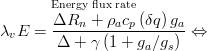$\overset{\text{Energy flux rate}}{\lambda_v E=\frac{\Delta R_n + \rho_a c_p \left( \delta q \right) g_a }{\Delta + \gamma \left ( 1 + g_a / g_s \right)}}\Leftrightarrow$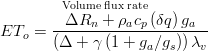$\overset{\text{Volume flux rate}}{ET_o=\frac{\Delta R_n + \rho_a c_p \left( \delta q \right) g_a } { \left( \Delta + \gamma \left ( 1 + g_a / g_s \right) \right) \lambda_v }}$

λv = Latent heat of vaporization. Energy required per unit mass of water vaporized. (J/g)
Lv = Volumetric latent heat of vaporization. Energy required per water volume vaporized. (Lv = 2453 MJ m-3)
E = Mass water evapotranspiration rate (g s-1 m-2)
ETo = Water volume evapotranspired (m3 s-1 m-2)
Δ = Rate of change of saturation specific humidity with air temperature. (Pa K-1)
Rn = Net irradiance (W m-2), the external source of energy flux
cp = Specific heat capacity of air (J kg-1 K-1)
ρa = dry air density (kg m-3)
δe = vapor pressure deficit, or specific humidity (Pa)
ga = Hydraulic conductivity of air, atmospheric conductance (m s-1)
gs = Conductivity of stoma, surface conductance (m s-1)
γ = Psychrometric constant (γ ≈ 66 Pa K-1)

Mohid Land has a sub-daily time step. According with FAO-56 Hourly Time Step ET is calulated with the equation below. This is the equation used in Mohid-Land.$\ ET_o=\frac{ \left 0.408\Delta(R_n - G) + \gamma \frac{37}{T_h+273}u_2 \left ( e^{o}*T_h-e_a \right )} { \Delta + \gamma \left (1 + 0.34u_2) }$

ETo = reference evapotranspiration [mm hour-1]
Rn = net radiation at the grass surface [MJ m-2 hour-1]
G = soil heat flux density [MJ m-2 hour-1]
Th = mean hourly air temperature at 2m hight [°C]
Δ = saturation slope vapour pressure curve at Th [kPa °C-1]
γ = psychrometric constant [kPa °C-1]
e°(Th) = saturation vapour pressure at air temperature Th [kPa]
ea = average hourly actual vapour pressure [kPa]
u2 = average hourly wind speed at 2m hight [m s-1].

Calculate Psicrometric constant: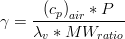$\gamma =\frac{ \left( c_p \right)_{air} * P }{ \lambda_v * MW_{ratio} }$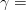$\gamma =$ psychrometric constant [kPa °C-1],
P = atmospheric pressure [kPa],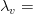$\lambda_v =$ latent heat of water vaporization, 2.45 [MJ kg-1],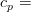$c_p =$ specific heat of air at constant pressure, 1.013 10-3 [MJ kg-1 °C-1],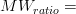$MW_{ratio} =$ ratio molecular weight of water vapor/dry air = 0.622.

Equation of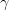$\gamma$ becomes: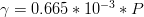$\gamma = 0.665*10^{-3} * P$

Calculation of the atmospheric pressure based on the heigth simplification of the ideal gas law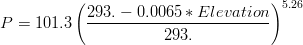$P = 101.3 \left ( \frac{293.-0.0065 * Elevation}{293.}\right ) ^{5.26}$

G beneath a dense cover of grass does not correlate well with air temperature. Hourly G can be approximated during daylight periods as: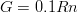$G = 0.1 Rn$

and during nighttime periods as: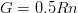$G = 0.5 Rn$

The net radiation (Rn) is the difference between the incoming net shortwave radiation (Rns) and the outgoing net longwave radiation (Rnl):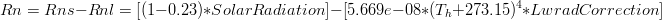$Rn = Rns - Rnl = [(1-0.23) * SolarRadiation] - [5.669e-08 * (T_h + 273.15)^{4} * LwradCorrection]$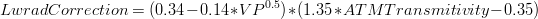$LwradCorrection = (0.34 - 0.14 * VP ^{0.5}) * (1.35 * ATMTransmitivity - 0.35)$

As saturation vapour pressure is related to air temperature, it can be calculated from the air temperature. The relationship is expressed by: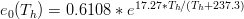$e_{0}(T_h) = 0.6108 * e^{17.27 * T_h / (T_h + 237.3)}$

The slope of the relationship between saturation vapour pressure and temperature: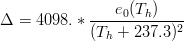$\Delta = 4098.* \frac {e_{0}(T_h)} {(T_h + 237.3)^{2}}$

The actual vapour pressure can also be calculated from the relative humidity.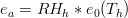$e_a = RH_h * e_{0}(T_h)$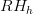$RH_h$ = Relative Humidity [-]

The Penman Montheith Potential Evapotranspiration computation will be active if in basin file

EVAPOTRANSPIRATION : 1


and the property evapotranspiration is not readed from file.

#### If the user is running with vegetation

than Crop Evapotranspiration is obtained from Potential Evapotranspiration using crop coefficient from Module Vegetation (dependent on crop).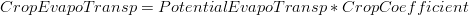$CropEvapoTransp = PotentialEvapoTransp * CropCoefficient$

Also if the user is running with vegetation a differentiation in Crop Evapotranspiration between Potential Transpiration and Potential Evaporation may be done using LAI:

EVAPOTRANSPIRATION_METHOD : 2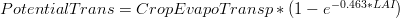$PotentialTrans = CropEvapoTransp * (1 - e^{-0.463 * LAI})$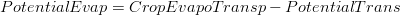$PotentialEvap = CropEvapoTransp - PotentialTrans$


if the user is running with vegetation and does not want the Crop Evapotranspiration to be separated in Potential Transpiration and Potential Evaporation then

EVAPOTRANSPIRATION_METHOD : 1


there will not be soil evaporation (in Module PorousMedia) and Crop Evapotranspiration is all transpiration (in Module Vegetation).

#### If the user is not running with vegetation

than Potential Evapotranspiration is all evaporation in the soil surface and will be done in Module PorousMedia.

#### Fluxes to other modules

If they are used, Potential transpiration computed in Module Basin is given to Module Vegetation to compute the effective transpiration. Potential Evaporation computed in Module Basin is given to Module Porous Media to compute the effective evaporation.

## Outputs

### .srb TimeSeries

water_column_[m] - runoff water column height in top of soil (height from soil surface)

water_level_[m] - runoff water altitude (in reference to mean sea level)

Infil._Rate_[mm/hour] - infiltration rate in the selected cell

Precipitation_Rate_[mm/hour] - precipitation rate in the selected cell

Throughfall_Rate_[mm/hour] - precipitation rate that arrives to soil surface (after leaf interception) in the selected cell

Canopy_Capacity_[m] - leaf capacity to accumulate water (in height) in the selected cell

Canopy_Storage_[m] - leaf accumulated water (in height) in the selected cell

Canopy_Drainage_[m] - leaf dip water (in height) in the selected cell

Reference_Evapotranspiration_[mm/h] - reference evapotranspiration (computed by Penman Motheith or given by the user)

Potential_Crop_EVTP_[mm/h] - reference evapotranspiration * Kc

Potential_Evaporation_[mm/h] - potential crop EVTP separated in evaporation (potential as may not occur depending on soil water availability)

Potential_Transpiration_[mm/h] - potential crop EVTP separated in transpiration (potential as may not occur depending on soil water availability)

EvapoTranspiration_Rate_[mm/hour] - actual evapotranspiration in the selected cell (the effevtive water removed)

Actual_Evaporation_[mm/h] - effective evaporation removed (from potential evaporation) - in surface soil

Actual_Transpiration_[mm/h] - effective transpiration removed (from potential transpiration) - along vegetation roots

### WaterBalance TimeSeries

Basin as the module that controls all others is also the responsible for computing mass balances (for water and properties)

There is a file with properties in m3 (Basin Water Balance) and for simplifying computation of acumulated mass balances there is other in m3/s (Basin Water Balance Fluxes).

Basin Water Balance Fluxes
Evap_From_Vegetation_m3/s               - evaporation from leafs
Evap_From_Ground_m3/s                   - evaporation from runoff water column
Evap_From_Soil_m3/s                     - evaporation from soil
Rain_Above_Leafs_m3/s                   - total rain
Rain_Uncovered_m3/s                     - rain in uncovered areas
Rain_Covered_m3/s                       - rain in leafs
Leaf_Drainage_m3/s                      - water that drains from leafs to runoff water column
Rain_Below_Leafs_m3/s                   - amount of rain uncovered + leaf drainage
Out_Volume_Channel_m3/s                 - volume that exits watershed in river – outlet and evaporation
Out_Volume_Overland_m3/s                - volume that exits watershed in runoff (if boundary condition on water can exit to outside, else no exit)
Infiltration_m3/s                       - water that infiltrates in soil
OL_Volume_To_Channels_m3/s              - flow from runoff to river (positive from runoff to river, negative from river to runoff)
GW_Volume_To_Channels_m3/s              - flow from porous media to river (positive from porousmedia to river, negative from river to porousmedia)


The accumulation in the watershed would be a difference between the inputs to the watershed and outputs from the watershed Accumulation = Sum ((In – Out)*LocalTimeStep) In -> Rain (Rain_Above_Leafs) + Discharges IN (there is no global output of the discharges, if you have input discharges you have to compute it with the values inserted) Out-> Water loss from soil or surface - Evapotranspiration (Evap_From_Vegetation + Evap_From_Ground + Evap_From_Soil) plus water lost from river (Out_Volume_Channel) pus water lost from runoff (Out_Volume_Overland)

Note: Negative discharges in river or discharges in runoff are not yet accounted in the mass balance (for mass error results). All that discharges have to be accounted if doing the accumulation computation yourself.